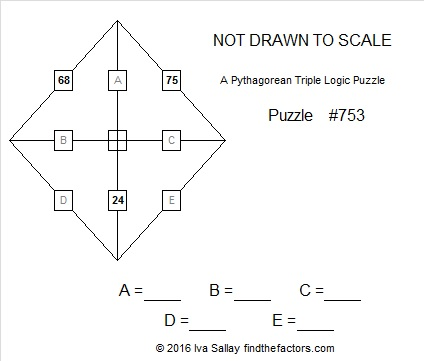# 753 Pythagorean Triple Puzzle

• 753 is a composite number.
• Prime factorization: 753 = 3 x 251
• The exponents in the prime factorization are 1 and 1. Adding one to each and multiplying we get (1 + 1)(1 + 1) = 2 x 2 = 4. Therefore 753 has exactly 4 factors.
• Factors of 753: 1, 3, 251, 753
• Factor pairs: 753 = 1 x 753 or 3 x 251
• 753 has no square factors that allow its square root to be simplified. √753 ≈ 27.440845.PUZZLE DIRECTIONS: This puzzle is NOT drawn to scale. Angles that are marked as right angles are 90 degrees, but any angle that looks like a 45 degree angle, isn’t 45 degrees. Lines that look parallel are NOT parallel. Shorter looking line segments may actually be longer than longer looking line segments. Most rules of geometry do not apply here: in fact non-adjacent triangles in the drawing might actually overlap.

No geometry is needed to solve this puzzle. All that is needed is the table of Pythagorean triples under the puzzle. The puzzle only uses triples in which each leg and each hypotenuse is less than 100 units long. The puzzle has only one solution.Print the puzzles or type the solution on this excel file: 12 Factors 2016-01-25—————————————

Here are some fun facts about the number 753:

753 is made from three consecutive odd numbers so it is divisible by 3.

753 can be written as the sum of consecutive numbers three ways:

• 376 + 377 = 753; that’s 2 consecutive numbers.
• 250 + 251 + 252 = 753; that’s 3 consecutive numbers.
• 123 + 124 + 125 + 126 + 127 + 128 = 753; that’s 6 consecutive numbers.

753 can be written as the sum of three cubes four ways, two of which duplicate squares:

• 25² + 8² + 8² = 753
• 22² + 13² + 10² = 753
• 20² + 17² + 8² = 753
• 19² + 14² + 14² = 753

From OEIS.org we learn that 753^3 = 426,957,777, the smallest positive perfect cube to contain 4 consecutive 7’s.

753 is palindrome 353 in BASE 15; note that 3(225) + 5(15) + 3(1) = 753.

—————————————

This site uses Akismet to reduce spam. Learn how your comment data is processed.